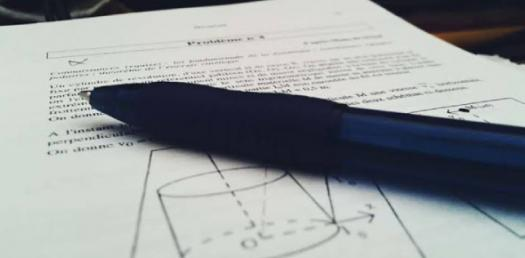# Hardest Physics Trivia Questions Test: Can You Pass The Quiz?

44 Questions | Attempts: 51
ShareSettingsBelow is the hardest physics trivia questions test, can you pass it? Do you think that you have done some extensive reading deserving the best grades when it comes to any quiz you may come across when it is about physics? How about you take up the quiz below and see if you might need more study hours?

• 1.
_______: Law of intertia
• 2.
_______: Changes in angular momentum
• 3.
_______: mg
• 4.
_______: Required for an object to float
• 5.
_______: mvr
• 6.
_______: Mass x velocity
• 7.
_______: F = ma
• 8.
_______: Conservation law requiring the absence of an unbalanced torque
• 9.
_______: Action and reaction
• 10.
_______: Capable of producing motion or a change in motion
• 11.
_______: Universal constant
• 12.
_______: SI unit of force
• 13.
_______: Resistance of relative motion
• 14.
_______: A nonzero vector sum of forces
• 15.
_______: Occurs in the absence of an unbalanced force
• 16.
_______: Describes the force of gravity
• 17.
_______: A measure of inertia
• 18.
_______: Gives the magnitude of the buoyant force
• 19.
What is the state of an object being acted upon by an unbalanced force?
• A.

At rest

• B.

In motion with a constant velocity

• C.

Accelerated

• D.

Zero speed

• 20.
What is a possible state of an object in the absence of a net force?
• A.

At rest

• B.

Constant speed

• C.

Zero acceleration

• D.

All of these

• 21.
What term refers to the tendency of an object to remain at rest or in uniform, straight-line motion?
• A.

Mass

• B.

Force

• C.

Inertia

• D.

All of these

• 22.
What is necessary for a change in velocity?
• A.

Inertia

• B.

An unbalance force

• C.

A zero net force

• D.

A change in direction

• 23.
A change in which of the following affects the weight of an object?
• A.

Momentum

• B.

Accelration due to gravity

• C.

Unbalanced force

• D.

A change in velocity

• 24.
For every action force, there is which of the following?
• A.

A net force

• B.

A friction force

• C.

An unbalanced force

• D.

An equal and opposite force

• 25.
Which is true of the force pair of Newton's third law?
• A.

The two forces never prodice an acceleartion.

• B.

The two forces act on different objects.

• C.

The two forces always cancel each other.

• D.

Newton's third law exists on for internal forces.

## Related TopicsBack to top
×

Wait!
Here's an interesting quiz for you.